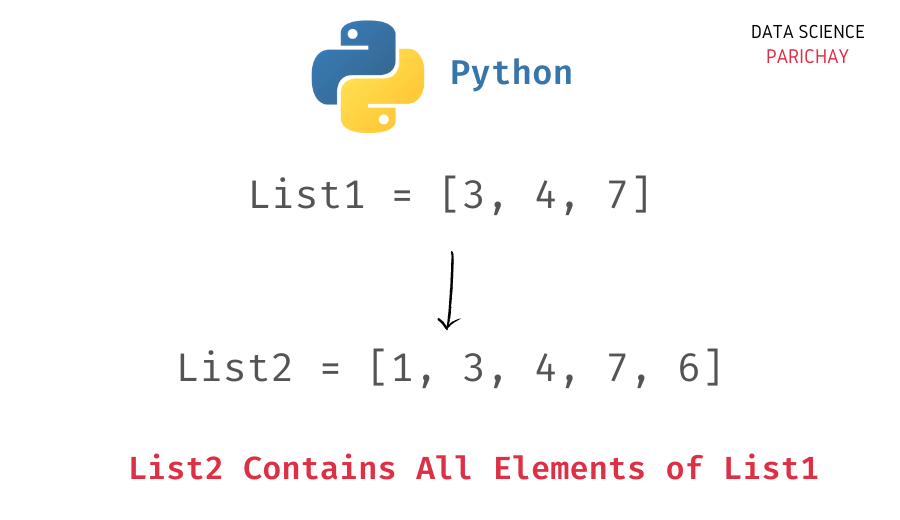# Python – Check List Contains All Elements Of Another List

In this tutorial, we will look at how to check if a list contains all the elements of another list in Python with the help of some examples.

In Python, you can use a combination of the built-in `all()` function, list comprehension, and the membership operator to check if a list contains all elements of another list.

Use the following steps to check if all elements in the list `ls1` are present in the list `ls2`

• In a list comprehension, for each element in the list `ls1`, check if it present in the list `ls2` using the membership operator, `in`.
• Apply the Python built-in `all()` function to return `True` only if all the items in the above list comprehension correspond to `True`.

You can use the following syntax to implement the above steps (check if all elements in `ls1` are present in `ls2`).

`all([item in ls2 for item in ls1])`

It returns `True` only if all the in `ls1` are present in `ls2`.

Let’s now look at some examples –

```# create two lists
ls1 = [1, 2, 3]
ls2 = [1, 2, 3, 4, 5]
# check if ls2 contains all elements from ls1
print(all([item in ls2 for item in ls1]))```

Output:

`True`

We get `True` as the output since all the elements in the list `ls1` are present in the list `ls2`.

📚 Data Science Programs By Skill Level

Introductory

Intermediate ⭐⭐⭐

🔎 Find Data Science Programs 👨‍💻 111,889 already enrolled

Disclaimer: Data Science Parichay is reader supported. When you purchase a course through a link on this site, we may earn a small commission at no additional cost to you. Earned commissions help support this website and its team of writers.

Let’s now look at another example.

```# create two lists
ls1 = [1, 2, 6]
ls2 = [1, 2, 3, 4, 5]
# check if ls2 contains all elements from ls1
print(all([item in ls2 for item in ls1]))```

Output:

`False`

Here we get `False` as the output because not all elements in the list `ls1` are present in the list `ls2`. The element `6` is not present in `ls2`.

Alternatively, you can convert the two lists to sets and use the set `issubset()` function to check if all elements in one list are present in another list or not.

```# create two lists
ls1 = [1, 2, 3]
ls2 = [1, 2, 3, 4, 5]
# check if ls2 contains all elements from ls1
s1 = set(ls1)
s2 = set(ls2)
print(s1.issubset(s2))```

Output:

`True`

We get the same result as above. Here, we convert the lists `ls1` and `ls2` to sets `s1` and `s2` respectively. And then check if the set `s1` is a subset of the set `s2`. This gives us whether all elements in `ls1` are present in `ls2` or not.

You might also be interested in –

•